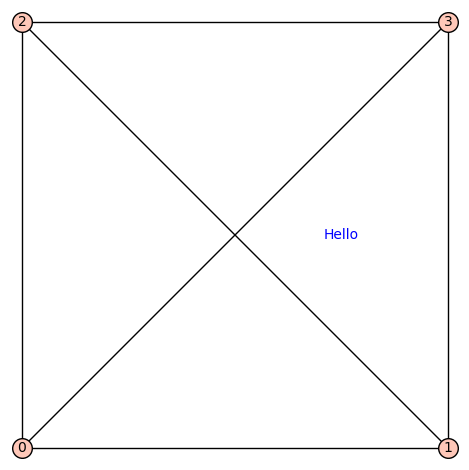# How can I add arbitrary text to a Graph object

I have created a Graph image such as shown belowusing the commands:

D = Graph(E)
D.show(pos=posd,**GPopt)


I would like to add text or a legend (explaining the colors) to the graph, but I have not found a way to add additional text to the Graph object. Suggestions welcome.

edit retag close merge delete

sage: sage.plot.graphics.Graphics.show? tells you that there is the "title" option. And also about "set_legend_options".

Thanks, that's a good pointer. The "title" and title_pos" options allows me to add a single text label. But what if I would like multiple text options? Although .show() recognizes "show legend", Graph does not accept "legend_label". I would expect that there is a general way of adding multiple text to a Graph such as D += text("hello world",(1,1))

Add a node having your text as label, no frame, no edges and manually positioned ?

The mode would still show as a node with the same format that the other nodes.

Sort by » oldest newest most votedTo add text to a plot, literally add the result of a call to text to the graphics object returned by plot:

sage: G = Graph([(0,1),(0,2),(0,3),(1,2),(1,3),(2,3)])
sage: G.plot(pos={ 0: (0,0), 1 : (1,0), 2: (0,1), 3: (1,1) },axes=False) + text("Hello",(0.75, 0.5))more

1

This works with G.plot() instead of G.show(), and answers my question. Thanks.

You're welcome. You can accept an answer by clicking the ✅

Thanks to rburing (above) here's a successful example of adding multiple text objects in different colors to a graph:more